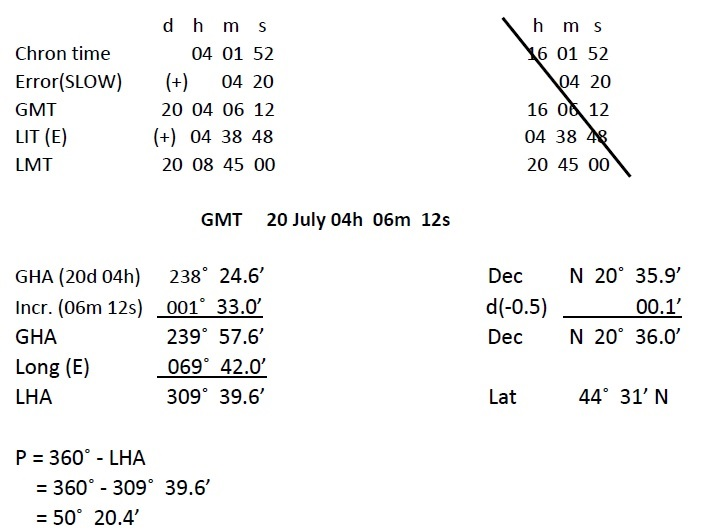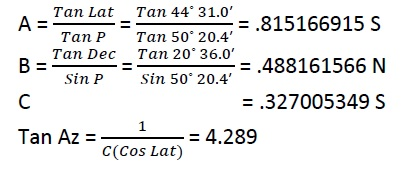# EXERCISE 28 — AZIMUTH SUN (Numerical Solution)

1. ###### On 20th July 2008, AM at ship in DR 44˚ 31’ N 069˚ 42’ E, the azimuth of the sun was 100˚(C) when chron showed 04h 01m 52s. If the chron error was 04m 20s SLOW and variation was 8˚E, find the deviation for the ship’s head.###### NOTE:
1. If LHA<180, P = LHA and If LHA>180, P = 360-LHA
2. Naming of A, B, C: A is named opposite to the latitude when LHA is between 270 & 90 and same as latitude when LHA is between 90 & 270. B is named same as declination. For C, if A & B are of same names, add and retain names. If of contrary names  then subtract and retain name of larger one.

We know that:###### NOTES:

Naming of Azimuth:  The prefix N or S is the name same as C whereas suffix E or W depends on the value of LHA. If LHA is between 0 to 180, the body lies to the WEST and if it is between 180 and 360, the body lies to the EAST.

Azimuth = S 76.9˚E
T Az= 103.1˚ (T)
C Az = 100.0˚ (C)
Error = 3.1˚ E
Variation = 8.0˚ E
Deviation= 4.9˚ W

###### NOTE:

The calculation of deviation is elementary chartwork. It can be easily calculated by referring the following simple formulae: Error – Variation = Deviation

Where, if error & variation are of same names then subtract and retain name. If of contrary names, then add and retain the name of the larger one.

1. ###### On 22nd Sept 2008, PM at ship in DR 18˚ 20’ N 085˚ 40’ E, the azimuth of the sun was 265˚(C) when the GPS clock showed 10h 09m 38s. If variation was 2˚W, calculate the deviation of the compass.

d     h     m      s

GMT                   22    10    09    38
LIT (E)                 05    42    40
LMT                    22   15     52     18